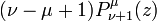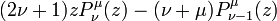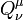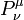# User:Tohline/Math/EQ Toroidal04

(Difference between revisions)
- [[Image:LSU_Key.png|25px|link=http://www.vistrails.org/index.php/User:Tohline/Appendix/Equation_templates#Special_Function_Relationships]] + [[Image:LSU_Key.png|25px|link=http://www.vistrails.org/index.php/User:Tohline/Appendix/Equation_templates#Relationships_Between_Various_Associated_Legendre_Functions]] - $~(\nu - \mu + 1)P^\mu_{\vu + 1}$ + $~(\nu - \mu + 1)P^\mu_{\nu + 1} (z)$ Line 13: Line 13: $~ [itex]~ - e^{i \mu \pi} ~ (2\pi)^{-\frac{1}{2}} (z^2-1)^{\mu/2} ~\Gamma(\mu + \tfrac{1}{2})~\biggl\{ + (2\nu + 1)z P_\nu^\mu(z) - (\nu + \mu)P^\mu_{\nu-1}(z) - \int_0^\pi (z - \cos t)^{-\mu - \frac{1}{2}} \cos[(\nu + \tfrac{1}{2})t] ~dt + - -\cos(\nu\pi) \int_0^\infty (z + \cosh t)^{-\mu - \frac{1}{2}} e^{-(\nu + \frac{1}{2})t} ~dt + - \biggr\} +$ [/itex]
- NOTE:  Both $~P_\nu^\mu$ and $~Q_\nu^\mu$ satisfy this same recurrence relation. + NOTE:  $~Q_\nu^\mu$, as well as $~P_\nu^\mu$, satisfies this same recurrence relation.$~(\nu - \mu + 1)P^\mu_{\nu + 1} (z)$$~=$$~ (2\nu + 1)z P_\nu^\mu(z) - (\nu + \mu)P^\mu_{\nu-1}(z)$ Abramowitz & Stegun (1995), p. 334, eq. (8.5.3)
 NOTE:$~Q_\nu^\mu$, as well as$~P_\nu^\mu$, satisfies this same recurrence relation.